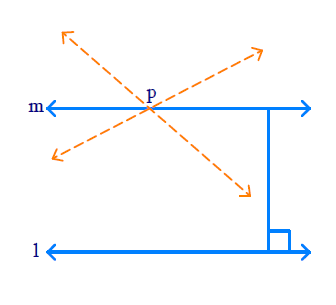In the verge of coronavirus pandemic, we are providing FREE access to our entire Online Curriculum to ensure Learning Doesn't STOP!

# Ex.5.2 Q1 Introduction to Euclids Geometry Solution - NCERT Maths Class 9

Go back to  'Ex.5.2'

## Question

How would you rewrite Euclid’s fifth postulate so that it would be easier to understand?

Video Solution
Introduction To Euclids Geometry
Ex 5.2 | Question 1

## Text Solution

steps:

For every line ‘$$l$$’ and for every point ‘$$P$$’ not lying on ‘$$l$$’, there exist a unique line $$‘m'$$ passing through ‘$$P$$’ and parallel to ‘$$l$$’.

This is called as ‘Playfair’s Axiom’• $$l$$ ’ is a line and ‘$$P$$ ’ is a point not lying on ’$$l$$ ’.
• We can draw infinite lines through ‘$$P$$ ’ but there is only one line unique which is parallel to ‘$$l$$ ’ and passes through ‘$$P$$ ’.
• Take any point on ‘$$l$$ ’ and draw to $$‘m'$$. Measure these distances.
• We know that it is same everywhere so these lines ‘$$l$$ ’ and $$‘m'$$ do not meet anywhere.

Learn from the best math teachers and top your exams

• Live one on one classroom and doubt clearing
• Practice worksheets in and after class for conceptual clarity
• Personalized curriculum to keep up with school